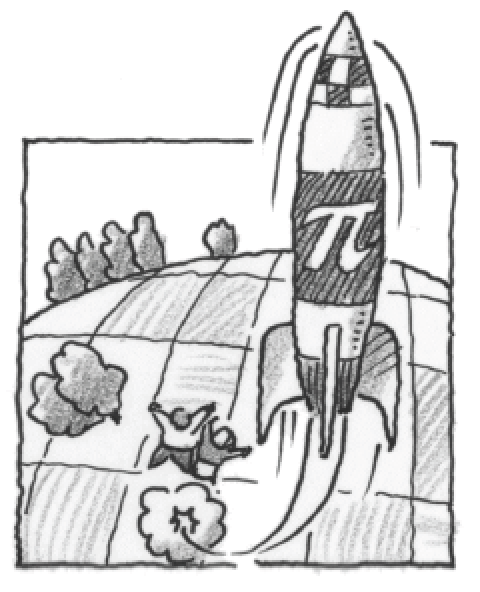### Home > APCALC > Chapter 5 > Lesson 5.1.1 > Problem5-9

5-9.The Math Booster Club shot a rocket from the ground straight up into the air to celebrate Pi Day. The launcher shot the rocket with a starting velocity of $182$ ft/sec. Write a function, $s$, for the height of the rocket at time, $t$. Then determine the rocket’s maximum height and the amount of time it was in the air.

Start with the basic equation: $s(t)=−16t^2+v_0t+s_0$ where $v_0$ is the initial velocity and $s_0$ is the initial height of the rocket from the ground.

The initial height of the rocket is $0$ because it is shot from the ground.

The initial velocity is $182$ ft/sec. Thus: $s(t) = −16t^2 + 182t$

To find the maximum height, take the derivative of the function and set it equal to zero because the maximum height occurs when the velocity is zero.

$0 = −32t +182$